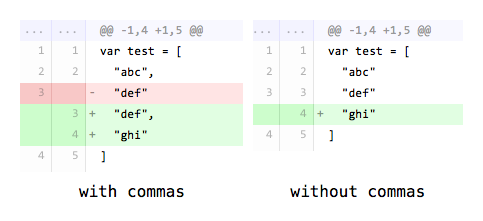# Compilation by a Thousand Semicolons and Commas

## 27 September 2015

Something I like in most modern programming languages is that they tend to ditch semicolons completely.

Semicolons are a nuisance, a waste of time, a source of (easily fixable) errors, ugly and unnecessary.

Compilers can easily work without them. Keeping them in old languages is, most of the time, simply a question of backward-compatibility.

And that's fine, after all. In modern languages though, this is something that must be avoided. It's wrong and useless.

Consider this snippet of Swift:

``````var greeting = "Hello!"

if let name = optionalName {
greeting = "Hello, \(name)"
}``````

No semicolon. Great. Now, consider a similar snippet of Go:

``````func sqrt(x float64) string {
if x < 0 {
return sqrt(-x) + "i"
}

return fmt.Sprint(math.Sqrt(x))
}``````

It's pretty nice, isn't it?

Go does use semicolons in its grammar. However, it's the lexer's role to add them, not the developer. Let the machines do the shit work.

Here's the extract from Effective Go:

Like C, Go's formal grammar uses semicolons to terminate statements, but unlike in C, those semicolons do not appear in the source. Instead the lexer uses a simple rule to insert semicolons automatically as it scans, so the input text is mostly free of them.

And unlike in an extremely popular language, Go imposes some formatting rules to its programmers and nobody has a problem with ASI.

However, there's still one perfectly valid case where semicolons are not optional:

``````if v := math.Pow(x, n); v < limit {
return v
}``````

Between `math.Pow(x, n)` and `v < limit`, you will find a semicolon. Because the two statements are on the same line, the compiler needs a semicolon to know where to end a statement and start the next one.

Basically, the rule is simple:

• Do not use a semicolon when a statement ends with a newline.
• Use a semicolon when a statement is followed by another statement on the same line.

That's the basic consensus in new languages.

So, here's my question: why don't we do the same thing with commas?!

Let's look at the following snippet of Swift:

``var test = ["a", "b", "c"]``

As you can see, each value of the array is separated by a comma. Same rule as the semicolons: if you are on the same line, use a separator. In this case, it's a comma instead of the semicolon because the semantic is different. Fine.

Now, let's look at the same snippet with a multi-lines notation — this example might seem contrived, but this kind of notation is clearer when the values are more complex and/or numerous:

``````var example = [
"a",
"b",
"c"
]``````

Wait. What. It's exactly like in the one-line declaration just above. Which is not surprising, because almost any language will do that.

That's inconsistent though. For semicolons, we omit them when there's a newline, but here, for a comma, we do not.

The correct syntax should be:

``````var test = [
"a"
"b"
"c"
]``````

Simpler, prettier. And it gives a few bonuses beyond the cosmetic aspect:

1. It's less error-prone.
2. It's more practical for versioning tool's diffs:3. It's consistent with the semicolon behavior.

I would apply the same rule to functions definition, maps or anything which needs commas, like I wrote in this example:

``````// One-line, comma.
func test(x: Int, y: Int) {}

// Or:

// Multi-lines, no comma.
func test(
x: Int
y: Int
) {

}``````

In Go, those notations are not frequent because the formatting rules are stricter. However, in a struct definition, you must write this:

``````type Point struct {
X int,
Y int,
}``````

(Note the trailing comma on the last line.)

It's better than what Swift does (at least, diffs are not impacted and you don't have to think to add or remove a comma — you just have to).

But why did they choose to keep the commas? No comma at all would have been way better AND coherent with the semicolon rule.

And you know what is even more inconsistent? This, which is perfectly valid Go code:

``````var (
x = 1
y = 2
z = 3
)``````

Yep, this time, there is nothing at all.